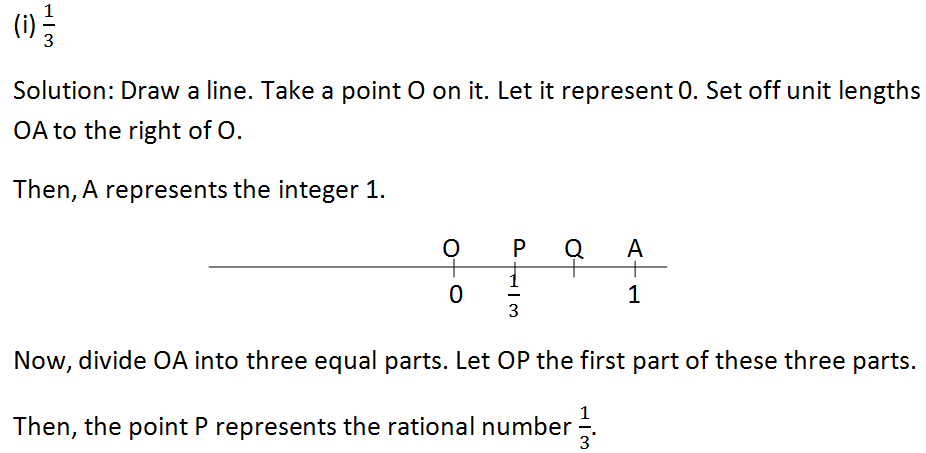# RS Aggarwal Class 7 Mathematics Fourth Chapter Rational Numbers Exercise 4B Solution

## EXERCISE 4B

(1) Represent each of the following rational numbers on the number line:(2) Which of the two rational numbers is greater in each of the following pairs?(3) Which of the two rational numbers is greater in each of the following pairs?(4) Fill in the blanks with the correct symbol out of >, = and <:(5) Arrange the following rational numbers in ascending order:(6) Arrange the following rational numbers in descending order:(7) Which of the following statements are true?(8) Find five rational numbers between -3 and -2.(9) Find five rational numbers between -1 and 1.Updated: May 30, 2022 — 1:05 pm